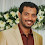# Bunker Calculation, Formula With Example And Sample Excel Sheet

Bunker calculation having very much significance in bunkering, at times wrong calculation can lead to shortage of bunker, oil spill etc. Here you can see  a few simple and convenient ways to calculate the bunker Quantity. You can also download the excel sheet for bunker calculation.
Bunker quantity is always said in Metric Ton (MT) because volume of oil changes according to the temperature , so it is always preferable to take bunker in weight which does not get changed with the change in temperature. But in actual scenario it's impossible to weigh the fuel oil physically . so we convert weight in to volume (m3).

To convert from MT to M3 we have to use the following basic formula

Volume(M3) = mass ( MT)/ density

But here the density is , "corrected density" ie, density of oil at the temperature of barge or terminal is pumping

Corrected Density= D(1-0.000645(T-15))

D= density at 15°C, this you will get from bunker advice form
T= Temperature of the fuel oil at which the barge or terminal is pumping.

Find the corrected density put into the basic formula ie,

Volume(M3) = mass ( MT)/ corrected density

From this you will get the total volume of bunker.

Go to your sounding table go to the page of your particular tank which you are planing to take bunker, find out the volume by considering the trim.  There you will get the final ullage or sounding of your tank.

Must Read> Bunker Delivery Note Details

Bunker calculation example:

Consider 285MT of HFO is planning to take on No.1 HFO port tank having capacity of 400m3 at trim of 0.50m.

Information you will get from bunker advice form,
Density at 15°C =0.9909
Temperature of fuel oil at the time of pumping. =50 Deg. C

Corrected density =D(1-0.000645(t-15))
=0.9909 ( 1-000645(50-15))

= 0.9682
Volume (M3)  = Mass (MT)/ corrected density
= 285/0.9682

= 294.35M3

Go to your sounding table for No.1 HFO port tank find 294.35M3   there you will get the final ullage or sounding.  Below you can see the diagram

>> Discussion is an exchange of knowledge It Make the Mariner Perfect.... Please Discuss below...

1.Great One simple Explained Nicely ...

1.2.Quantity in m3= 294,35 m3

Quantity * VCF * WCF= 294,35*0,9760*0,9898=284,36 MT

So with your calculation we have stortage

1.quantity in MT= quantity M3* corrected density
= 294.35*0.9682=285

3.hi can u give me some of example on how to calculate..using trim..temp.dencity

1.Already I have exemplified at the end of the article.. if you specify the area which is doubtful for you I shall explain it..

2.how would you get density @15f for oil store on land over a period of time at a powerplant

4.Where did you get .000645?

1.this is a correction factor used in bunker density calculation.

5.very nice program of your bunker caculation thank u sir

6.Nice formula easy to understand

1.thanks... visit again

7.8.9.Using 0.000645 for correcting density is an approximation because density does not change uniformly.It gives near correct value. ASTM tables will have to be used for calculating accurate quantity.

1.Explain me about bunker valve settings and transfer of bunker in two different tanks in same time .

2.For every relative density there is an equivalent coefficient of expansion.

10.Very nice.
So, If you have 1000 m3 f.o density at 15 C is 0.960
How can I calculate volume correction factor in temperature at 100 C.?

1.According to your data VCF may be 0.9388 found by Table 54-B.

11.Well explained sir

12.What is the basic to learn to become a bunker surveyor? Please guide me as im about to hired soon. Thank you

1.i m not in that field... But generally saying, bunker surveyor's duty is to independently check and determine the barge delivered quantity and the vessel received quantity. These two quantities should be within acceptable tolerance.... My advice is just google it and have some acquire some general knowledge about bunker surveying.... Any way the company who hired you definitely train you for the job.... If you have a good communication skills and mediating skills then this job wont be a big deal for you.... any way lets see somebody will answer your question better way...

13.Is the 0.000645 corrective factor for fuel? do you have MDO corr. factor?

1.you can use same correction factor for DO also

2.For DO 0.00011

14.From where can I get max bunker intake flow rate ?

1.Maximum bunker intake flow rate can be seen on your ship's bunker documents. Usually you can check it in the previous bunker checklist which shows the maximum intake rate and pressure at the manifold.

15.Kindly illustrate bunker calculation along with using VCF, WCF, Corrected ullage/sounding, list, heel, trim correction.

16.R.O.B – Bunker calculation

Interpolate to find the corresponding value for average sounding 3.30 m=179.2 m3,3.40 m=183.4 m3. Find the X value?

Find the mass of fuel oil

17.Length= 34m